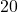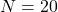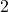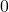# C++ Program for Sum of Even and Odd Numbers

This is a simple program to compute the sum of first 10 even and odd numbers and display the results.It is intended for beginner level learners of C programming.

The program is written and compiled using Dev-C++ 4.9.9.2 version compiler tool. You will find following sections in this post – problem definition, flowchart, program source code and verified output to help you understand and learn C programming language.  Try to practice writing, compiling and run this program on your own.

## Problem Definition

The program for computing sum of first 10 even and odd numbers follow very simple logic, given below

1. Take firstnumber,of which half are even and half are odd numbers.
2. Check each number one at a time and see if it’s divisible by.
3. If Yes, add the number to Sum_Even.
4. Else, add the number to Sum_Odd.
5. Display the results
6. End the program

Note:- Whenever a number is divisible byit gives a remainder of, otherwise not.

## Program Code – Sum of Even and Odd

//Program to Compute Sum of first 10 Even and First 10 Odd Numbers

#include <iostream.h>
#include <stdlib.h>
#include <stdio.h>

int main()
{
int sum_even,sum_odd,N,i;

//Initialize the sum of even and sum of odd numbers
sum_even = 0;
sum_odd = 0;

// Total of 20 numbers comprise of 10 odd and 10 even numbers
// But you change the value of N to anything if required
N = 20;

//Compute the sum of even and sum of odd number
for(i = 1;i<=N;i++)
{
if((i % 2) == 0)
{
sum_even = sum_even + i;
}
else
{
sum_odd = sum_odd + i;
}
}

//Print the results now
for(i=0;i<35;i++)
printf("_"); printf("\n\n");

cout <<; "Th sum of 10 evens are"
<< "\t" << sum_even << endl;

cout << "The sum of 10 odd are"
<<"\t" << sum_odd << endl;

for(i=0;i<35;i++)
printf("_"); printf("\n\n");

getch();
return 0;
}

## Output

The output of the program is given below. Remember that the program does ask for input because the value of N is already assigned. You can change that to a program asking input at run-time.

                           _______________________________________
Sum of First 10 Even Numbers:       110
Sum of First 10 Odd Numbers:        100
_______________________________________